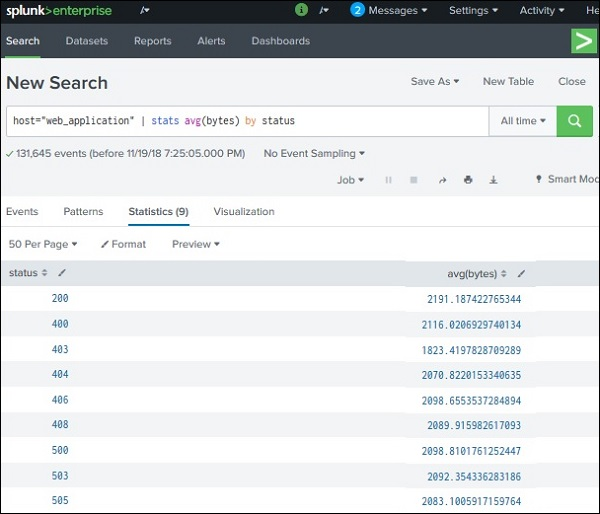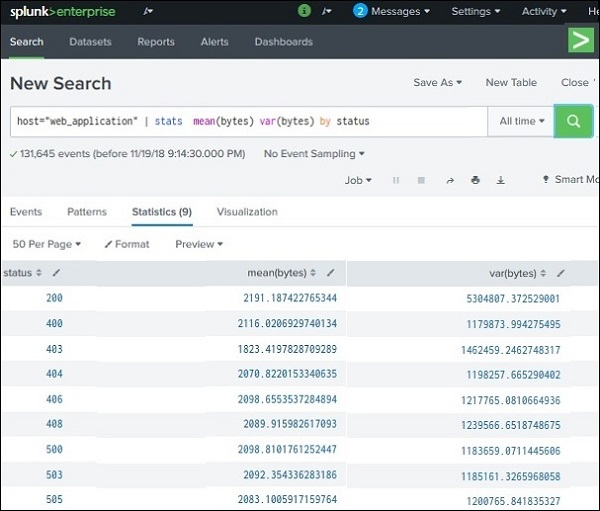# Splunk - Stats Command

The stats command is used to calculate summary statistics on the results of a search or the events retrieved from an index. The stats command works on the search results as a whole and returns only the fields that you specify.

Each time you invoke the stats command, you can use one or more functions. However, you can only use one BY clause. If the stats command is used without a BY clause, only one row is returned, which is the aggregation over the entire incoming result set. If a BY clause is used, one row is returned for each distinct value specified in the BY clause.

Below we see the examples on some frequently used stats command.

## Finding Average

We can find the average value of a numeric field by using the avg() function. This function takes the field name as input. Without a BY clause, it will give a single record which shows the average value of the field for all the events. But with a by clause, it will give multiple rows depending on how the field is grouped by the additional new field.

In the below example, we find the average byte size of the files grouped by the various http status code linked to the events associated with those files.## Finding Range

The stats command can be used to display the range of the values of a numeric field by using the range function. We continue the previous example but instead of average, we now use the max(), min() and range function together in the stats command so that we can see how the range has been calculated by taking the difference between the values of max and min columns.## Finding Mean and Variance

Statistically focused values like the mean and variance of fields is also calculated in a similar manner as given above by using appropriate functions with the stats command. In the below example, we use the functions mean() & var() to achieve this. We continue using the same fields as shown in the previous examples. The result shows the mean and variance of the values of the field named bytes in rows organized by the http status values of the events.